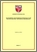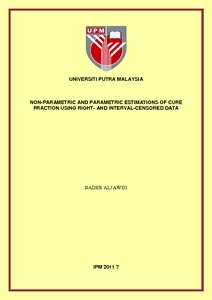Simple Search:

# Non-Parametric and Parametric Estimations of Cure Fraction Using Right-and Interval-Censored Data

## Citation

Aljawdi, Bader (2011) Non-Parametric and Parametric Estimations of Cure Fraction Using Right-and Interval-Censored Data. PhD thesis, Universiti Putra Malaysia.

## Abstract / Synopsis

In cancer studies many patients will never experience the event of concern and are hence considered as cured. Therefore, the main interest of the cancer trials is in determining the fraction of cured patients so as to obtain the trends in the survival of cancer patients. Survival models incorporate the cure fraction in the analysis; namely cure rate models, are being widely used in cancer studies, where one of the most common cure models that can be employed in cancer clinical trials is the bounded cumulative hazard (BCH) model. In this thesis, we considered two methods via the expectation maximization (EM) algorithm for cure rate estimation based on the BCH model using the two censoring types common to cancer clinical trials; namely, right and interval censoring. Then, a series of simulation studies was conducted to evaluate the performance of the proposed estimation approaches. This research investigated the non-parametric maximum likelihood estimation method for cure rate estimation by considering two common estimators for the survival function: 1) The Kaplan Meier (KM) estimator, which is suitable for the right censoring case; and 2) The Turnbull Estimator, which is suitable for the interval type of data censoring. The parametric maximum likelihood estimation of the cure fraction was also investigated under the same circumstances considering two scenarios: 1) when covariates were excluded from the analysis. In this case, the estimation was developed based on the exponential and Weibull distributions using the right and interval censoring types; and 2) when covariates were incorporated into the analysis through the scaleparameter of the exponential distribution only using the same types of data censoring. The major research findings were as follows: 1) the non-parametric and parametric estimation methods using the right and interval censoring types produced highly efficient cure rate parameters when the censoring rate was decreased to the minimum possible; 2) Non-parametric estimation of the cure fraction using interval censored data based on Turnbull estimator resulted in more precise cure fraction than the Kaplan Meier estimator considering the interval midpoint to represent the exact life time; 3) The parametric estimation of the cure fraction based on the exponential distribution and right and interval censoring types produced more consistent estimates than the Weibull distribution especially in case of heavy censoring; 4) Parametric estimation of the cure fraction was more efficient when some covariates had been involved in the analysis than when covariates had been excluded; and 5) the nonparametric estimation method is the viable alternative to the parametric one when the data set contains substantial censored observations while in the case of low censoring the parametric method is more attractive.

## Download FilePreview
PDF
IPM_2011_7.pdf

Download (448kB) | Preview

## Additional Metadata

Item Type: Thesis (PhD) Fractional calculus Expectation-maximization algorithms Estimation theory IPM 2011 7 Institute for Mathematical Research Haridan Mohd Jais 19 Mar 2014 12:33 19 Mar 2014 12:33 http://psasir.upm.edu.my/id/eprint/19694 View Download Statistic

### Actions (login required)View Item